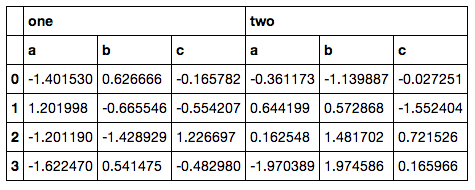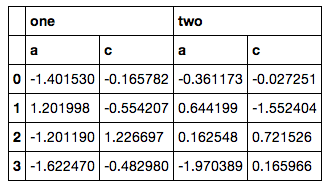# Selecting columns from pandas MultiIndex

I have DataFrame with MultiIndex columns that looks like this:

```# sample data
col = pd.MultiIndex.from_arrays([['one', 'one', 'one', 'two', 'two', 'two'],
['a', 'b', 'c', 'a', 'b', 'c']])
data = pd.DataFrame(np.random.randn(4, 6), columns=col)
data```What is the proper, simple way of selecting only specific columns (e.g. `['a', 'c']`, not a range) from the second level?

Currently I am doing it like this:

```import itertools
tuples = [i for i in itertools.product(['one', 'two'], ['a', 'c'])]
new_index = pd.MultiIndex.from_tuples(tuples)
print(new_index)
data.reindex_axis(new_index, axis=1)```It doesn’t feel like a good solution, however, because I have to bust out `itertools`, build another MultiIndex by hand and then reindex (and my actual code is even messier, since the column lists aren’t so simple to fetch). I am pretty sure there has to be some `ix` or `xs` way of doing this, but everything I tried resulted in errors.

Contents

### Method 1

The most straightforward way is with `.loc`:

```>>> data.loc[:, (['one', 'two'], ['a', 'b'])]

one       two
a    b    a    b
0  0.4 -0.6 -0.7  0.9
1  0.1  0.4  0.5 -0.3
2  0.7 -1.6  0.7 -0.8
3 -0.9  2.6  1.9  0.6```

Remember that `[]` and `()` have special meaning when dealing with a `MultiIndex` object:

(…) a tuple is interpreted as one multi-level key

(…) a list is used to specify several keys [on the same level]

(…) a tuple of lists refer to several values within a level

When we write `(['one', 'two'], ['a', 'b'])`, the first list inside the tuple specifies all the values we want from the 1st level of the `MultiIndex`. The second list inside the tuple specifies all the values we want from the 2nd level of the `MultiIndex`.

Edit 1: Another possibility is to use `slice(None)` to specify that we want anything from the first level (works similarly to slicing with `:` in lists). And then specify which columns from the second level we want.

```>>> data.loc[:, (slice(None), ["a", "b"])]

one       two
a    b    a    b
0  0.4 -0.6 -0.7  0.9
1  0.1  0.4  0.5 -0.3
2  0.7 -1.6  0.7 -0.8
3 -0.9  2.6  1.9  0.6```

If the syntax `slice(None)` does appeal to you, then another possibility is to use `pd.IndexSlice`, which helps slicing frames with more elaborate indices.

```>>> data.loc[:, pd.IndexSlice[:, ["a", "b"]]]

one       two
a    b    a    b
0  0.4 -0.6 -0.7  0.9
1  0.1  0.4  0.5 -0.3
2  0.7 -1.6  0.7 -0.8
3 -0.9  2.6  1.9  0.6```

When using `pd.IndexSlice`, we can use `:` as usual to slice the frame.

### Method 2

It’s not great, but maybe:

```>>> data
one                           two
a         b         c         a         b         c
0 -0.927134 -1.204302  0.711426  0.854065 -0.608661  1.140052
1 -0.690745  0.517359 -0.631856  0.178464 -0.312543 -0.418541
2  1.086432  0.194193  0.808235 -0.418109  1.055057  1.886883
3 -0.373822 -0.012812  1.329105  1.774723 -2.229428 -0.617690
>>> data.loc[:,data.columns.get_level_values(1).isin({"a", "c"})]
one                 two
a         c         a         c
0 -0.927134  0.711426  0.854065  1.140052
1 -0.690745 -0.631856  0.178464 -0.418541
2  1.086432  0.808235 -0.418109  1.886883
3 -0.373822  1.329105  1.774723 -0.617690```

would work?

### Method 3

You can use either, `loc` or `ix` I’ll show an example with `loc`:

`data.loc[:, [('one', 'a'), ('one', 'c'), ('two', 'a'), ('two', 'c')]]`

When you have a MultiIndexed DataFrame, and you want to filter out only some of the columns, you have to pass a list of tuples that match those columns. So the itertools approach was pretty much OK, but you don’t have to create a new MultiIndex:

`data.loc[:, list(itertools.product(['one', 'two'], ['a', 'c']))]`

### Method 4

I think there is a much better way (now), which is why I bother pulling this question (which was the top google result) out of the shadows:

`data.select(lambda x: x in ['a', 'b'], axis=1)`

gives your expected output in a quick and clean one-liner:

```        one                 two
a         b         a         b
0 -0.341326  0.374504  0.534559  0.429019
1  0.272518  0.116542 -0.085850 -0.330562
2  1.982431 -0.420668 -0.444052  1.049747
3  0.162984 -0.898307  1.762208 -0.101360```

It is mostly self-explaining, the `` refers to the level.

### Method 5

#### `ix` and `select` are deprecated!

The use of `pd.IndexSlice` makes `loc` a more preferable option to `ix` and `select`.

#### `DataFrame.loc` with `pd.IndexSlice`

```# Setup
col = pd.MultiIndex.from_arrays([['one', 'one', 'one', 'two', 'two', 'two'],
['a', 'b', 'c', 'a', 'b', 'c']])
data = pd.DataFrame('x', index=range(4), columns=col)
data

one       two
a  b  c   a  b  c
0   x  x  x   x  x  x
1   x  x  x   x  x  x
2   x  x  x   x  x  x
3   x  x  x   x  x  x```
```data.loc[:, pd.IndexSlice[:, ['a', 'c']]]

one    two
a  c   a  c
0   x  x   x  x
1   x  x   x  x
2   x  x   x  x
3   x  x   x  x```

You can alternatively an `axis` parameter to `loc` to make it explicit which axis you’re indexing from:

```data.loc(axis=1)[pd.IndexSlice[:, ['a', 'c']]]

one    two
a  c   a  c
0   x  x   x  x
1   x  x   x  x
2   x  x   x  x
3   x  x   x  x```

#### `MultiIndex.get_level_values`

Calling `data.columns.get_level_values` to filter with `loc` is another option:

```data.loc[:, data.columns.get_level_values(1).isin(['a', 'c'])]

one    two
a  c   a  c
0   x  x   x  x
1   x  x   x  x
2   x  x   x  x
3   x  x   x  x```

This can naturally allow for filtering on any conditional expression on a single level. Here’s a random example with lexicographical filtering:

```data.loc[:, data.columns.get_level_values(1) > 'b']

one two
c   c
0   x   x
1   x   x
2   x   x
3   x   x```

More information on slicing and filtering MultiIndexes can be found at Select rows in pandas MultiIndex DataFrame.

### Method 6

To select all columns named `'a'` and `'c'` at the second level of your column indexer, you can use slicers:

```>>> data.loc[:, (slice(None), ('a', 'c'))]

one                 two
a         c         a         c
0 -0.983172 -2.495022 -0.967064  0.124740
1  0.282661 -0.729463 -0.864767  1.716009
2  0.942445  1.276769 -0.595756 -0.973924
3  2.182908 -0.267660  0.281916 -0.587835```

### Method 7

A slightly easier, to my mind, riff on Marc P.‘s answer using slice:

```import pandas as pd
col = pd.MultiIndex.from_arrays([['one', 'one', 'one', 'two', 'two', 'two'], ['a', 'b', 'c', 'a', 'b', 'c']])
data = pd.DataFrame(np.random.randn(4, 6), columns=col)

data.loc[:, pd.IndexSlice[:, ['a', 'c']]]

one                 two
a         c         a         c
0 -1.731008  0.718260 -1.088025 -1.489936
1 -0.681189  1.055909  1.825839  0.149438
2 -1.674623  0.769062  1.857317  0.756074
3  0.408313  1.291998  0.833145 -0.471879```

As of pandas 0.21 or so, .select is deprecated in favour of .loc.

### Method 8

Use `df.loc(axis="columns")` (or `df.loc(axis=1)` to access just the columns and slice away:

`df.loc(axis="columns")[:, ["a", "c"]]`

### Method 9

The .loc[:, list of column tuples] approach given in one of the earlier answers fails in case the multi-index has boolean values, as in the example below:

```col = pd.MultiIndex.from_arrays([[False, False, True,  True],
[False, True,  False, True]])
data = pd.DataFrame(np.random.randn(4, 4), columns=col)
data.loc[:,[(False, True),(True, False)]]```

This fails with a `ValueError: PandasArray must be 1-dimensional.`

Compare this to the following example, where the index values are strings and not boolean:

```col = pd.MultiIndex.from_arrays([["False", "False", "True",  "True"],
["False", "True",  "False", "True"]])
data = pd.DataFrame(np.random.randn(4, 4), columns=col)
data.loc[:,[("False", "True"),("True", "False")]]```

This works fine.

You can transform the first (boolean) scenario to the second (string) scenario with

```data.columns = pd.MultiIndex.from_tuples([(str(i),str(j)) for i,j in data.columns],
names=data.columns.names)```

and then access with string instead of boolean column index values (the `names=data.columns.names` parameter is optional and not relevant to this example). This example has a two-level column index, if you have more levels adjust this code correspondingly.

Getting a boolean multi-level column index arises, for example, if one does a crosstab where the columns result from two or more comparisons.

### Method 10

Two answers are here depending on what is the exact output that you need.

If you want to get a one leveled dataframe from your selection (which can be sometimes really useful) simply use :

`df.xs('theColumnYouNeed', level=1, axis=1)`

If you want to keep the multiindex form (similar to metakermit’s answer) :

`data.loc[:, data.columns.get_level_values(1) == "columnName"]`

Hope this will help someone